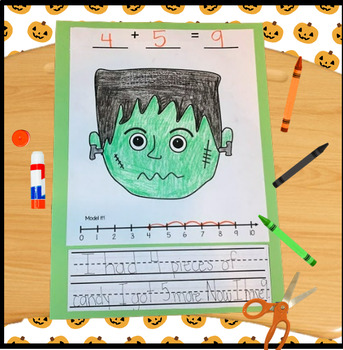# Frankenstein Math CraftSubject
Resource Type
File Type

PDF

(674 KB)
Standards
• Product Description
• StandardsNEW

Hey there teacher,

Here is a great Halloween math activity for your students! Students can create their own addition or subtraction Frankenstein problem. They are asked to write the equation, draw a model, and use a number line. If you want to extend the activity you can have kids write out the story problem. If you enjoyed this please stop by my store to leave feedback. Thanks and happy teaching!

-Bee A Learner

Apply properties of operations as strategies to add and subtract. If 8 + 3 = 11 is known, then 3 + 8 = 11 is also known. (Commutative property of addition.) To add 2 + 6 + 4, the second two numbers can be added to make a ten, so 2 + 6 + 4 = 2 + 10 = 12. (Associative property of addition.)
Solve word problems that call for addition of three whole numbers whose sum is less than or equal to 20, e.g., by using objects, drawings, and equations with a symbol for the unknown number to represent the problem.
Use addition and subtraction within 20 to solve word problems involving situations of adding to, taking from, putting together, taking apart, and comparing, with unknowns in all positions, e.g., by using objects, drawings, and equations with a symbol for the unknown number to represent the problem.
Fluently add and subtract within 5.
For any number from 1 to 9, find the number that makes 10 when added to the given number, e.g., by using objects or drawings, and record the answer with a drawing or equation.
Total Pages
N/A
N/A
Teaching Duration
N/A
Report this Resource to TpT
Reported resources will be reviewed by our team. Report this resource to let us know if this resource violates TpT’s content guidelines.